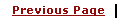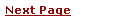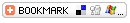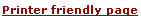C - Basic DatatypesLearning C
C Function References
C Useful Resources# C - Basic DatatypesC has a concept of 'data types' which are used to define a variable before its use. The definition of a variable will assign storage for the variable and define the type of data that will be held in the location.

The value of a variable can be changed any time.

C has the following basic built-in datatypes.

• int

• float

• double

• char

Please note that there is not a boolean data type. C does not have the traditional view about logical comparison, but thats another story.

## int - data type

int is used to define integer numbers.

 ``` { int Count; Count = 5; } ```

## float - data type

float is used to define floating point numbers.

 ``` { float Miles; Miles = 5.6; } ```

## double - data type

double is used to define BIG floating point numbers. It reserves twice the storage for the number. On PCs this is likely to be 8 bytes.

 ``` { double Atoms; Atoms = 2500000; } ```

## char - data type

char defines characters.

 ``` { char Letter; Letter = 'x'; } ```

# Modifiers

The data types explained above have the following modifiers.

• short
• long
• signed
• unsigned

The modifiers define the amount of storage allocated to the variable. The amount of storage allocated is not cast in stone. ANSI has the following rules:

 ``` short int <= int <= long int float <= double <= long double ```

What this means is that a 'short int' should assign less than or the same amount of storage as an 'int' and the 'int' should be less or the same bytes than a 'long int'. What this means in the real world is:

 ``` Type Bytes Range --------------------------------------------------------------------- ``` ``` short int 2 -32,768 -> +32,767 (32kb) unsigned short int 2 0 -> +65,535 (64Kb) unsigned int 4 0 -> +4,294,967,295 ( 4Gb) int 4 -2,147,483,648 -> +2,147,483,647 ( 2Gb) long int 4 -2,147,483,648 -> +2,147,483,647 ( 2Gb) signed char 1 -128 -> +127 unsigned char 1 0 -> +255 float 4 double 8 long double 12 ```

These figures only apply to todays generation of PCs. Mainframes and midrange machines could use different figures, but would still comply with the rule above.

You can find out how much storage is allocated to a data type by using the sizeof operator discussed in Operator Types Session.

Here is an example to check size of memory taken by various datatypes.

 ```int main() { printf("sizeof(char) == %d\n", sizeof(char)); printf("sizeof(short) == %d\n", sizeof(short)); printf("sizeof(int) == %d\n", sizeof(int)); printf("sizeof(long) == %d\n", sizeof(long)); printf("sizeof(float) == %d\n", sizeof(float)); printf("sizeof(double) == %d\n", sizeof(double)); printf("sizeof(long double) == %d\n", sizeof(long double)); printf("sizeof(long long) == %d\n", sizeof(long long)); return 0; } ```

# Qualifiers

A type qualifier is used to refine the declaration of a variable, a function, and parameters, by specifying whether:

• The value of a variable can be changed.
• The value of a variable must always be read from memory rather than from a register

Standard C language recognizes the following two qualifiers:

• const
• volatile

The const qualifier is used to tell C that the variable value can not change after initialisation.

const float pi=3.14159;

Now pi cannot be changed at a later time within the program.

Another way to define constants is with the #define preprocessor which has the advantage that it does not use any storage

The volatile qualifier declares a data type that can have its value changed in ways outside the control or detection of the compiler (such as a variable updated by the system clock or by another program). This prevents the compiler from optimizing code referring to the object by storing the object's value in a register and re-reading it from there, rather than from memory, where it may have changed. You will use this qualifier once you will become expert in "C". So for now just proceed.

# What are Arrays:

We have seen all baisc data types. In C language it is possible to make arrays whose elements are basic types. Thus we can make an array of 10 integers with the declaration.

 ```int x; ```

The square brackets mean subscripting; parentheses are used only for function references. Array indexes begin at zero, so the elements of x are:

Thus Array are special type of variables which can be used to store multiple values of same data type. Those values are stored and accessed using subscript or index.

Arrays occupy consecutive memory slots in the computer's memory.

 ```x, x, x, ..., x ```

If an array has n elements, the largest subscript is n-1.

Multiple-dimension arrays are provided. The declaration and use look like:

 ``` int name ; n = name[i+j]  + name[k] ; ```

Subscripts can be arbitrary integer expressions. Multi-dimension arrays are stored by row so the rightmost subscript varies fastest. In above example name has 10 rows and 20 columns.

Same way, arrays can be defined for any data type. Text is usually kept as an array of characters. By convention in C, the last character in a character array should be a `\0' because most programs that manipulate character arrays expect it. For example, printf uses the `\0' to detect the end of a character array when printing it out with a `%s'.

Here is a program which reads a line, stores it in a buffer, and prints its length (excluding the newline at the end).

 ``` main( ) { int n, c; char line; n = 0; while( (c=getchar( )) != '\n' ) { if( n < 100 ) line[n] = c; n++; } printf("length = %d\n", n); } ```

## Array Initialization

• As with other declarations, array declarations can include an optional initialization
• Scalar variables are initialized with a single value
• Arrays are initialized with a list of values
• The list is enclosed in curly braces
 ```int array  = {2, 4, 6, 8, 10, 12, 14, 16}; ```

The number of initializers cannot be more than the number of elements in the array but it can be less in which case, the remaining elements are initialized to 0.if you like, the array size can be inferred from the number of initializers by leaving the square brackets empty so these are identical declarations:

 ```int array1  = {2, 4, 6, 8, 10, 12, 14, 16}; int array2 [] = {2, 4, 6, 8, 10, 12, 14, 16}; ```

An array of characters ie string can be initialized as follows:

 ```char string = "Hello"; ```